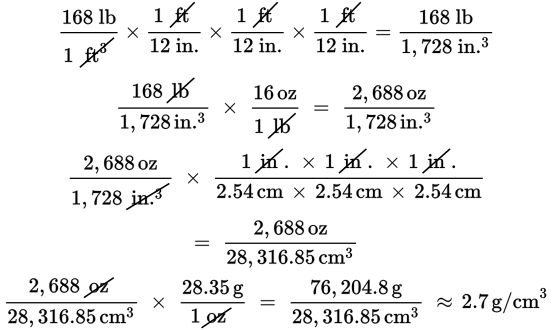# SAT Math Multiple Choice Question 449: Answer and Explanation

### Test Information

Question: 449

14. A scientist weighed a 1.0 cubic foot piece of granite and found that it weighed 168 pounds. The average density of Earth's inner core is approximately 12.8 g/cm3. How much denser, in g/cm3, is Earth's inner core than the piece of granite? Use any of the following conversions:

12 inches = 1 foot

16 ounces = 1 pound

1 inch = 2.54 cm

1 ounce = 28.35 grams

• A. 2.7
• B. 10.1
• C. 15.55
• D. 28.35

Explanation:

B

Difficulty: Hard

Category: Problem Solving and Data Analysis / Rates, Ratios, Proportions, and Percentages

Strategic Advice: Let the units in this question guide you to the correct answer. Set up unit conversions so that the units you don't want will cancel out. Your goal is to find the density of the piece of granite so you can compare it to the density of Earth's inner core. You don't need a definition of density—you can see from the units that you need to convert to g/cm3, which tells you to divide the weight of the granite by the volume.

Getting to the Answer: You're not given a conversion from feet to centimeters or pounds to grams, so you'll need to convert 168 pounds per cubic foot to ounces per cubic inch, and then to grams per cubic centimeter. Try not to round too much as you work—rather, wait until the very end if possible.But wait—that's not the final answer. Subtract this amount from 12.8 to find that Earth's inner core is 12.8 – 2.7 = 10.1 g/cm3 denser than the piece of granite.